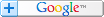## "Can Fibonacci Price Projections Forecast Key Turning Points In The Markets?..."es... and, with great accuracy. You'll see how calculations based on the Fibonacci number series can be applied to stock prices for startling results.Applied correctly, support and resistance levels will materialize. Likely turning points will present themselves, clearly.

So, how are the retracement percentages derived?

Begin by adding one to itself... which is two. Then add two to one to get three -- the next number in the series. You keep adding the current number to the previous number so it looks like this...

1, 1, 2, 3, 5, 8, 13, 21, 34, 55, 89, 144...

Now, you have a number series which goes on forever.Next, there is an important ratio which is calculated by dividing one number in the series by its previous number. For example...

144 ÷ 89 = 1.618

This ratio is known as the Golden Mean and is the basis for most retracement percentages. Equally important is the reciprocal of 1.618...

1 ÷ 1.618 = .618

Here are some other percentages you'll want to use...

.382 = .618 squared

.500 = 1 ÷ 2, the second and third numbers in the series

.786 = square root of .618

1.000 = 1.618 x .618

1.272 = square root of 1.618

2.618 = 1.618 squared### "How are Fibonacci percentages applied?"

Begin by locating isolated highs and lows in a trend. The various swing points will be used to measure the retracements.

In the following illustration, trend AB has been established...Counter-trend BC is a 50-percent retracement of trend AB. Trend CD continues in the same direction as trend AB. Counter-trend DE is a 38-percent retracement of trend CD.

Shallow retracements are typical in a strong trend.Combining retracements from multiple swing points is a dynamic forecasting method. In the next example, retracement percentages are calculated from trends AD and CD...The 38-percent retracement is calculated from trend AD. The 50-percent retracement is from trend CD. When retracements from different swing points cluster together it is known as confluence.

Areas of confluence are likely turning points.

Click For Fibonacci Confluence Chart ExamplesSo far, you have seen how to calculate probable retracements using the high and low swing point of an established trend. You can use Fibonacci percentages, in combination, to forecast the end of a trend... with excellent results.

This next example shows a typical five-wave trend progression where the 1.618 and .618 percentage extensions are used...First, trend AB is multiplied by 1.618 and then added to itself... forecasting a target price at point F. Next, counter-trend BC retraces part of trend AB before continuing on. Finally, trend AD is multiplied by .618 and added to itself. This projection also forecasts the trend termination at point F. Incredibly powerful!

In a five-wave pattern, this ratio combination will bear-out turning points, again and again.There are many other ways to apply Fibonacci retracements. Purchase the following books to learn more...by Robert Fischer
John Wiley & Sons, Inc.
2001
352 Pages

Get This Book, Now!Dynamic Trading - Dynamic Concepts in Time, Price and Pattern Analysis with Practical Strategies for Traders and Investors
by Robert C. Miner
1997
564 Pages

 Or... SEARCH for a BOOK Enter title, author, item# or ISBNHome | Charting Principles | Forecasting Tools | Trading Systems | Market Metrics

Charts In Action | Market Commentary | Online Newsletter | Bookstore

For the Record | Site MapOnline Newsletter Subscribe FREE! And... receive your FREE report. Back Issues ofChart WealthWhat's an RSS feed?